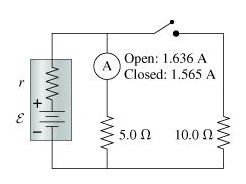# Problem: A) What is the emf of the battery in the figure ?B) What is the internal resistance of the battery in the figure?

###### FREE Expert Solution

Equivalent resistance for two resistors in parallel:

$\overline{){{\mathbf{R}}}_{\mathbf{e}\mathbf{q}}{\mathbf{=}}\frac{{\mathbf{R}}_{\mathbf{1}}{\mathbf{R}}_{\mathbf{2}}}{{\mathbf{R}}_{\mathbf{1}}\mathbf{+}{\mathbf{R}}_{\mathbf{2}}}}$

Current when the switch is open:

${\mathbit{i}}_{\mathbf{0}}\mathbf{=}\frac{\mathbf{\epsilon }}{\mathbf{r}\mathbf{+}{\mathbf{R}}_{\mathbf{5}\mathbf{.}\mathbf{0}}}$

1.636 = ε/(r + 5.0)

When the switch is closed, the current through the 5.0-Ω resistor is:

i1 = 1.565A

Voltage, V = i1R

V = i1(5.0) = (1.565)(5.0) = 7.825 V

The potential across the 10.0-Ω resistor is:

V = 7.825 V

Current across the 10.0-Ω resistor is:

i2 = 7.825/10.0 = 0.7825A

When teh switch is closed,

i = i1 + i2 = 1.565 + 0.7825 = 2.3475A

The resistors are in parallel:

Req = (5.0)(10.0)/(5.0 + 10.0) = 3.333Ω

99% (239 ratings)###### Problem DetailsA) What is the emf of the battery in the figure ?

B) What is the internal resistance of the battery in the figure?